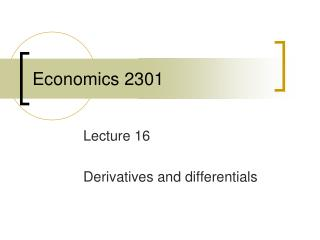# Economics 2301 - PowerPoint PPT PresentationDownload PresentationEconomics 2301

Economics 2301
Download Presentation## Economics 2301

- - - - - - - - - - - - - - - - - - - - - - - - - - - E N D - - - - - - - - - - - - - - - - - - - - - - - - - - -
##### Presentation Transcript

1. Economics 2301 Lecture 16 Derivatives and differentials

2. Differentiability • A function is differentiable over a certain interval if a derivative exists for each point in that interval. • Not all functions are differentiable in their entire domain. • The derivative can be thought of as the slope of a line tangent to the original function. If there is no unique tangent line at a certain value of a function, then a derivative does not exist for that value.

3. Figure 6.6 Functions Not Everywhere Differentiable

4. Differentiability A function is differentiable over a certain interval if each point in that interval is associated with a unique tangent line. This requires, in turn, that, in this interval, the function be continuous and smooth. A function is smooth if it has no “corner points,” that is, no points where it is possible to draw more than one tangent line.

5. Figure 6.7 Oligopolistic Demand and Cost Functions

6. Average and marginal functions • The simple geometry of the relationship between marginal and average compares the slope of a ray from the origin to the value of the derivative of a function as we move lout along the function. • The slope of the ray from the origin will increase as we move out along the function as long as the derivative of the function is greater than the slope of the ray from the origin.

7. Average and marginal functions • The slope of the ray from the origin will decrease as we move out along the function as long as the derivative is less than the slope of that ray. • A linear function has a constant slope, a constant derivative, and there, a unvarying average value.